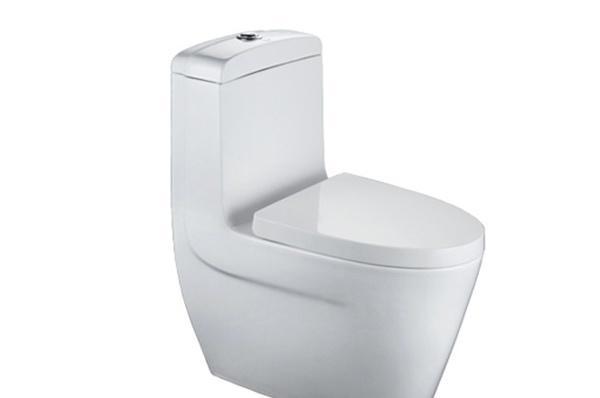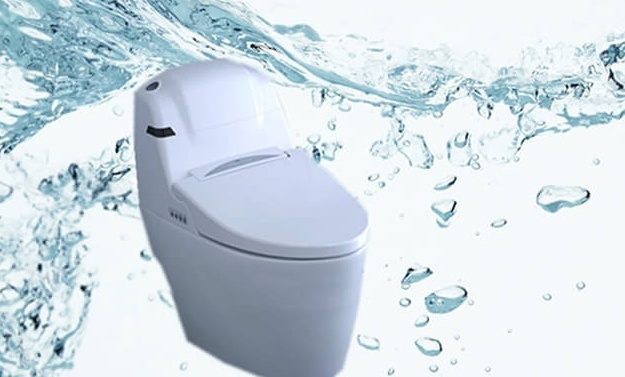|

# 中国十大马桶品牌有哪些

中国十大马桶品牌完全的为消费者提供了最可靠的参考依据，即使在购买的时候苯教难以选择，但可以通过参考介绍，来了解各个马桶品牌的基本信息。中国十大马桶品牌有哪些

创立于1917年日本，生产、销售民用及商业设施用卫浴及相关设备的企业，座便器十大品牌，东陶（中国）有限公司。 东陶公司是一个生产、销售民用及商业设施用卫浴、洁具及相关设备的厂家。追求高品质、高工艺水平，使用户享受卫生、健康、舒适的生活是公司一贯追求的目标。

东陶公司致力于保护珍贵的水资源，创造和保持舒适的生活环境，发展一体化组合结构的卫生设备产品，不断的增强自身产品实力，开拓着新的市场空间。

东陶公司应用“水与电子相结合”的工艺技术以及其它相关技术，创造出首屈一指的东陶产品。使TOTO的产品以其卓越的功能和极高的可靠性而着称于世。并且东陶“水与电子相结合”为基础的产品系列在同业界被公认为第一产家。

2.箭牌Arrow

中国驰名商标，中国名牌，生产卫浴洁具、瓷砖及配套产品的企业，马桶十大品牌，佛山市顺德区乐华陶瓷洁具有限公司。

3.惠达Huida

于1982年，中国驰名商标，中国名牌，中国500最具价值品牌，座便器十大品牌，唐山惠达陶瓷（集团）股份有限公司。

唐山惠达陶瓷集团始创于1982年，是中国规模最大，历史最悠久的卫浴家居用品企业之一。总部位于唐山惠达陶瓷城，员工总数近万人。产品种类超过2000多种。其中，洗面器、座便器等陶瓷洁具年产量为900万件，共有17条天然气隧道窑生产线。此外，惠达还拥有墙地砖、浴室柜、五金龙头及配件等卫浴家居配套产品。4、东鹏DONGPENG

东鹏洁具始创于1994年广东佛山，专注于卫浴的研发、制造、销售、服务的卫浴企业。产品线覆盖卫生陶瓷、浴室家具、休闲卫浴、智能卫浴、龙头五金、厨房五金等六大品类，为客户提供完善的整体卫浴空间解决方案。

5、浪鲸SSWW

浪鲸卫浴创始于1994年，专注卫浴行业20年，迄今已建成集设计、研发、生产、营销于一体的休闲卫浴和卫生陶瓷两大生产基地，以及专业产品检测中心。产品涵盖按摩缸、蒸汽房、淋浴房、洗手盆、坐便器、浴室柜、五金龙头等十多个品种，规模庞大。

6、航标BOLINA

航标卫浴为航标控股旗下品牌，创建于1997年，是专业制造卫生陶瓷的生产企业，为国家级高新技术企业。系列产品包含陶瓷卫生洁具、五金龙头挂件、浴缸、淋浴房、浴室柜等五大系列1000多款品种型号。

7、和成HCG

和成卫浴是和成集团旗下的核心品牌。和成于1931年创立于台湾，是全球着名卫浴厂商，享有“全球十大卫浴厂商”美誉，是中国大陆四大卫浴品牌之一，和成横跨建筑、精密陶瓷、炉具及住宅设备等多个领域。8、澳斯曼卫浴

澳斯曼卫浴创建于广东，作为中国卫浴的十大品牌之一，主打产品涉及卫浴家具、卫浴五金、坐便器等等，涉及范围广泛，澳斯曼卫浴先后获得过中国十大卫浴品牌等多个荣誉称号，现在已经发展成了中国卫浴的领导品牌，在同行业中也有着不少的支持和影响力。澳斯曼卫浴的质量和品质是消费者看在眼里的，更是在心里的。

9、法恩莎卫浴

成为中国卫浴十大品牌之一的法恩莎，其实力也是不容小觑的。在同行业中，是最具综合力的企业之一，主打产品具有广泛的知名度和影响力，能有这样的成就，和消费者的信赖是分不开的，更离不开法恩莎源源不断的追求，追求完美品质、高标准；法恩莎的产品极具时尚化，体现在制造商、工艺上、科技上这三个方面。

10、 安华卫浴

安华作为中国卫浴十大品牌之一，其上榜理由：第一，品牌质量保证，安华品牌涉及的产品主要就是卫浴产品这一块，还包括瓷砖、卫浴洁具等，作为一个知名品牌，安华产品当然都是只做最好的；第二、企业文化，企业文化虽然和产品没有太多的关联，但正是因为企业文化的理念和宗旨，去一步步的实现产品的生产，为消费者创造高品质卫浴生活。

`声明：本文由入驻焦点开放平台的作者撰写，除焦点官方账号外，观点仅代表作者本人，不代表焦点立场错误信息举报电话： 400-099-0099，邮箱：jubao@vip.sohu.com，或点此进行意见反馈，或点此进行举报投诉。`A B C D E F G H J K L M N P Q R S T W X Y Z
A - B - C - D - E
• A
• 鞍山
• 安庆
• 安阳
• 安顺
• 安康
• 澳门
• B
• 北京
• 保定
• 包头
• 巴彦淖尔
• 本溪
• 蚌埠
• 亳州
• 滨州
• 北海
• 百色
• 巴中
• 毕节
• 保山
• 宝鸡
• 白银
• 巴州
• C
• 承德
• 沧州
• 长治
• 赤峰
• 朝阳
• 长春
• 常州
• 滁州
• 池州
• 长沙
• 常德
• 郴州
• 潮州
• 崇左
• 重庆
• 成都
• 楚雄
• 昌都
• 慈溪
• 常熟
• D
• 大同
• 大连
• 丹东
• 大庆
• 东营
• 德州
• 东莞
• 德阳
• 达州
• 大理
• 德宏
• 定西
• 儋州
• 东平
• E
• 鄂尔多斯
• 鄂州
• 恩施
F - G - H - I - J
• F
• 抚顺
• 阜新
• 阜阳
• 福州
• 抚州
• 佛山
• 防城港
• G
• 赣州
• 广州
• 桂林
• 贵港
• 广元
• 广安
• 贵阳
• 固原
• H
• 邯郸
• 衡水
• 呼和浩特
• 呼伦贝尔
• 葫芦岛
• 哈尔滨
• 黑河
• 淮安
• 杭州
• 湖州
• 合肥
• 淮南
• 淮北
• 黄山
• 菏泽
• 鹤壁
• 黄石
• 黄冈
• 衡阳
• 怀化
• 惠州
• 河源
• 贺州
• 河池
• 海口
• 红河
• 汉中
• 海东
• 怀来
• I
• J
• 晋中
• 锦州
• 吉林
• 鸡西
• 佳木斯
• 嘉兴
• 金华
• 景德镇
• 九江
• 吉安
• 济南
• 济宁
• 焦作
• 荆门
• 荆州
• 江门
• 揭阳
• 金昌
• 酒泉
• 嘉峪关
K - L - M - N - P
• K
• 开封
• 昆明
• 昆山
• L
• 廊坊
• 临汾
• 辽阳
• 连云港
• 丽水
• 六安
• 龙岩
• 莱芜
• 临沂
• 聊城
• 洛阳
• 漯河
• 娄底
• 柳州
• 来宾
• 泸州
• 乐山
• 六盘水
• 丽江
• 临沧
• 拉萨
• 林芝
• 兰州
• 陇南
• M
• 牡丹江
• 马鞍山
• 茂名
• 梅州
• 绵阳
• 眉山
• N
• 南京
• 南通
• 宁波
• 南平
• 宁德
• 南昌
• 南阳
• 南宁
• 内江
• 南充
• P
• 盘锦
• 莆田
• 平顶山
• 濮阳
• 攀枝花
• 普洱
• 平凉
Q - R - S - T - W
• Q
• 秦皇岛
• 齐齐哈尔
• 衢州
• 泉州
• 青岛
• 清远
• 钦州
• 黔南
• 曲靖
• 庆阳
• R
• 日照
• 日喀则
• S
• 石家庄
• 沈阳
• 双鸭山
• 绥化
• 上海
• 苏州
• 宿迁
• 绍兴
• 宿州
• 三明
• 上饶
• 三门峡
• 商丘
• 十堰
• 随州
• 邵阳
• 韶关
• 深圳
• 汕头
• 汕尾
• 三亚
• 三沙
• 遂宁
• 山南
• 商洛
• 石嘴山
• T
• 天津
• 唐山
• 太原
• 通辽
• 铁岭
• 泰州
• 台州
• 铜陵
• 泰安
• 铜仁
• 铜川
• 天水
• 天门
• W
• 乌海
• 乌兰察布
• 无锡
• 温州
• 芜湖
• 潍坊
• 威海
• 武汉
• 梧州
• 渭南
• 武威
• 吴忠
• 乌鲁木齐
X - Y - Z
• X
• 邢台
• 徐州
• 宣城
• 厦门
• 新乡
• 许昌
• 信阳
• 襄阳
• 孝感
• 咸宁
• 湘潭
• 湘西
• 西双版纳
• 西安
• 咸阳
• 西宁
• 仙桃
• 西昌
• Y
• 运城
• 营口
• 盐城
• 扬州
• 鹰潭
• 宜春
• 烟台
• 宜昌
• 岳阳
• 益阳
• 永州
• 阳江
• 云浮
• 玉林
• 宜宾
• 雅安
• 玉溪
• 延安
• 榆林
• 银川
• Z
• 张家口
• 镇江
• 舟山
• 漳州
• 淄博
• 枣庄
• 郑州
• 周口
• 驻马店
• 株洲
• 张家界
• 珠海
• 湛江
• 肇庆
• 中山
• 自贡
• 资阳
• 遵义
• 昭通
• 张掖
• 中卫

1室1厅1厨1卫1阳台

1
2
3
4
5

0
1
2

1

1

0
1
2
3报名成功，资料已提交审核A B C D E F G H J K L M N P Q R S T W X Y Z
A - B - C - D - E
• A
• 鞍山
• 安庆
• 安阳
• 安顺
• 安康
• 澳门
• B
• 北京
• 保定
• 包头
• 巴彦淖尔
• 本溪
• 蚌埠
• 亳州
• 滨州
• 北海
• 百色
• 巴中
• 毕节
• 保山
• 宝鸡
• 白银
• 巴州
• C
• 承德
• 沧州
• 长治
• 赤峰
• 朝阳
• 长春
• 常州
• 滁州
• 池州
• 长沙
• 常德
• 郴州
• 潮州
• 崇左
• 重庆
• 成都
• 楚雄
• 昌都
• 慈溪
• 常熟
• D
• 大同
• 大连
• 丹东
• 大庆
• 东营
• 德州
• 东莞
• 德阳
• 达州
• 大理
• 德宏
• 定西
• 儋州
• 东平
• E
• 鄂尔多斯
• 鄂州
• 恩施
F - G - H - I - J
• F
• 抚顺
• 阜新
• 阜阳
• 福州
• 抚州
• 佛山
• 防城港
• G
• 赣州
• 广州
• 桂林
• 贵港
• 广元
• 广安
• 贵阳
• 固原
• H
• 邯郸
• 衡水
• 呼和浩特
• 呼伦贝尔
• 葫芦岛
• 哈尔滨
• 黑河
• 淮安
• 杭州
• 湖州
• 合肥
• 淮南
• 淮北
• 黄山
• 菏泽
• 鹤壁
• 黄石
• 黄冈
• 衡阳
• 怀化
• 惠州
• 河源
• 贺州
• 河池
• 海口
• 红河
• 汉中
• 海东
• 怀来
• I
• J
• 晋中
• 锦州
• 吉林
• 鸡西
• 佳木斯
• 嘉兴
• 金华
• 景德镇
• 九江
• 吉安
• 济南
• 济宁
• 焦作
• 荆门
• 荆州
• 江门
• 揭阳
• 金昌
• 酒泉
• 嘉峪关
K - L - M - N - P
• K
• 开封
• 昆明
• 昆山
• L
• 廊坊
• 临汾
• 辽阳
• 连云港
• 丽水
• 六安
• 龙岩
• 莱芜
• 临沂
• 聊城
• 洛阳
• 漯河
• 娄底
• 柳州
• 来宾
• 泸州
• 乐山
• 六盘水
• 丽江
• 临沧
• 拉萨
• 林芝
• 兰州
• 陇南
• M
• 牡丹江
• 马鞍山
• 茂名
• 梅州
• 绵阳
• 眉山
• N
• 南京
• 南通
• 宁波
• 南平
• 宁德
• 南昌
• 南阳
• 南宁
• 内江
• 南充
• P
• 盘锦
• 莆田
• 平顶山
• 濮阳
• 攀枝花
• 普洱
• 平凉
Q - R - S - T - W
• Q
• 秦皇岛
• 齐齐哈尔
• 衢州
• 泉州
• 青岛
• 清远
• 钦州
• 黔南
• 曲靖
• 庆阳
• R
• 日照
• 日喀则
• S
• 石家庄
• 沈阳
• 双鸭山
• 绥化
• 上海
• 苏州
• 宿迁
• 绍兴
• 宿州
• 三明
• 上饶
• 三门峡
• 商丘
• 十堰
• 随州
• 邵阳
• 韶关
• 深圳
• 汕头
• 汕尾
• 三亚
• 三沙
• 遂宁
• 山南
• 商洛
• 石嘴山
• T
• 天津
• 唐山
• 太原
• 通辽
• 铁岭
• 泰州
• 台州
• 铜陵
• 泰安
• 铜仁
• 铜川
• 天水
• 天门
• W
• 乌海
• 乌兰察布
• 无锡
• 温州
• 芜湖
• 潍坊
• 威海
• 武汉
• 梧州
• 渭南
• 武威
• 吴忠
• 乌鲁木齐
X - Y - Z
• X
• 邢台
• 徐州
• 宣城
• 厦门
• 新乡
• 许昌
• 信阳
• 襄阳
• 孝感
• 咸宁
• 湘潭
• 湘西
• 西双版纳
• 西安
• 咸阳
• 西宁
• 仙桃
• 西昌
• Y
• 运城
• 营口
• 盐城
• 扬州
• 鹰潭
• 宜春
• 烟台
• 宜昌
• 岳阳
• 益阳
• 永州
• 阳江
• 云浮
• 玉林
• 宜宾
• 雅安
• 玉溪
• 延安
• 榆林
• 银川
• Z
• 张家口
• 镇江
• 舟山
• 漳州
• 淄博
• 枣庄
• 郑州
• 周口
• 驻马店
• 株洲
• 张家界
• 珠海
• 湛江
• 肇庆
• 中山
• 自贡
• 资阳
• 遵义
• 昭通
• 张掖
• 中卫• 手机• 分享
• 设计
免费设计
• 计算器
装修计算器
• 入驻
合作入驻
• 联系
联系我们
• 置顶
返回顶部